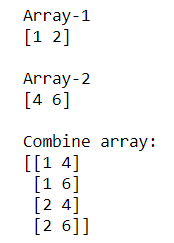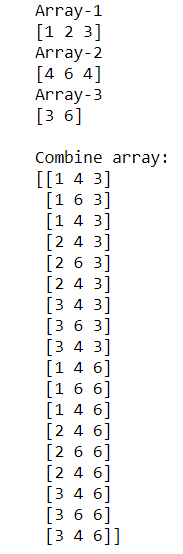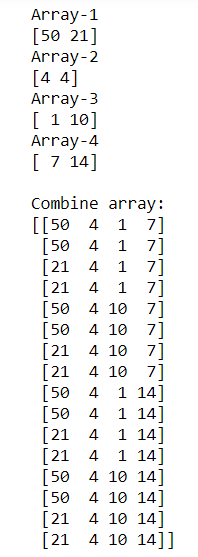# How to build an array of all combinations of two NumPy arrays?

Sometimes we need to find the combination of elements of two or more arrays. Numpy has a function to compute the combination of 2 or more Numpy arrays named as “numpy.meshgrid()“. This function is used to create a rectangular grid out of two given one-dimensional arrays representing the Cartesian indexing or Matrix indexing.

Syntax:

```numpy.meshgrid(*xi, copy=True, sparse=False, indexing='xy')
```

Example 1: Computing combinations of elements of Two NumPy arrays

## Python3

 `# importing Numpy package ` `import` `numpy as np ` ` `  `# creating 2 numpy arrays ` `array_1 ``=` `np.array([``1``, ``2``]) ` `array_2 ``=` `np.array([``4``, ``6``]) ` ` `  `print``(``"Array-1"``) ` `print``(array_1) ` ` `  `print``(``"\nArray-2"``) ` `print``(array_2) ` ` `  `# combination of elements of array_1 and array_2 ` `# using numpy.meshgrid().T.reshape() ` `comb_array ``=` `np.array(np.meshgrid(array_1, array_2)).T.reshape(``-``1``, ``2``) ` ` `  `print``(``"\nCombine array:"``) ` `print``(comb_array) `

Output:In the above example, we combine elements of ‘array_1‘ and ‘array_2‘ using numpy.meshgrid().T.reshape()

Example 2: Computing combinations of elements of Three NumPy arrays

## Python3

 `# importing Numpy package ` `import` `numpy as np ` ` `  `# creating 3 numpy arrays ` `array_1 ``=` `np.array([``1``, ``2``, ``3``]) ` `array_2 ``=` `np.array([``4``, ``6``, ``4``]) ` `array_3 ``=` `np.array([``3``, ``6``]) ` ` `  `print``(``"Array-1"``) ` `print``(array_1) ` ` `  `print``(``"Array-2"``) ` `print``(array_2) ` ` `  `print``(``"Array-3"``) ` `print``(array_3) ` ` `  ` `  `# combination of elements of array_1, ` `# array_2 and array_3 using  ` `# numpy.meshgrid().T.reshape() ` `comb_array ``=` `np.array( ` `  ``np.meshgrid(array_1, array_2, array_3)).T.reshape(``-``1``, ``3``) ` ` `  `print``(``"\nCombine array:"``) ` `print``(comb_array) `

Output:In the above example, we combine elements of ‘array_1‘, ‘array_2‘ and ‘array_3‘ using numpy.meshgrid().T.reshape()

Example 3: Computing combinations of elements of Four NumPy arrays

## Python3

 `# importing Numpy package ` `import` `numpy as np ` ` `  `# creating 4 numpy arrays ` `array_1 ``=` `np.array([``50``, ``21``]) ` `array_2 ``=` `np.array([``4``, ``4``]) ` `array_3 ``=` `np.array([``1``, ``10``]) ` `array_4 ``=` `np.array([``7``, ``14``]) ` ` `  ` `  `print``(``"Array-1"``) ` `print``(array_1) ` ` `  `print``(``"Array-2"``) ` `print``(array_2) ` ` `  `print``(``"Array-3"``) ` `print``(array_3) ` ` `  `print``(``"Array-4"``) ` `print``(array_4) ` ` `  ` `  `# combination of elements of array_1,  ` `# array_2, array_3 and array_4 ` `# using numpy.meshgrid().T.reshape() ` `comb_array ``=` `np.array(np.meshgrid( ` `    ``array_1, array_2, array_3, array_4)).T.reshape(``-``1``, ``4``) ` ` `  `print``(``"\nCombine array:"``) ` `print``(comb_array) `

Output:In the above example, we combine elements of ‘array_1‘, ‘array_2‘, ‘array_3‘ and ‘array_4‘ using numpy.meshgrid().T.reshape()

Attention geek! Strengthen your foundations with the Python Programming Foundation Course and learn the basics.

To begin with, your interview preparations Enhance your Data Structures concepts with the Python DS Course.

My Personal Notes arrow_drop_upCheck out this Author's contributed articles.

If you like GeeksforGeeks and would like to contribute, you can also write an article using contribute.geeksforgeeks.org or mail your article to contribute@geeksforgeeks.org. See your article appearing on the GeeksforGeeks main page and help other Geeks.

Please Improve this article if you find anything incorrect by clicking on the "Improve Article" button below.

Article Tags :

Be the First to upvote.

Please write to us at contribute@geeksforgeeks.org to report any issue with the above content.